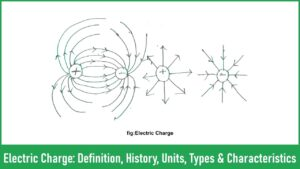# Electric Charge: Definition, History, Units, Types & Characteristics

## What is an electric charge?Electric charge is defined as the source of the electric field because charges are responsible for creating an electric field. Material like electron, proton, and neutron having some basic properties apart from their masses, and these additional properties create an electrical force are called electric charge. The charge can not be differentiated using shape, size, color, fluidity efficiency.
Electric charge is an important property of electrostatics. Cells or capacitors can store electric charge.

### What is a point charge?

When the sizes or dimensions of any charged bodies are very small as compared to the distances between them (where its effect are studied), it is called point charges. In space, all the charge content of the body is considered to be concentrated at one point.

### What is a charged body?

A material or body can gain or exit electrons. A material or body can excess of electrons of deficit of electrons as compared to a neutral body is called a charged body.

## History of electric charge

Historically around 600 BC at Thales of Miletus of Greece, a substance is found named as Ambar Which when rubbed with wool or silk it attracts the object from where the concept of charge is developed.
The scientist William Gilbert first derived the idea of electric charge. The concept of electric charge derived from the substance Ambar which has a strong charge acquiring property.

## Types Of Electric Charge

Du fay shows there are two kinds of electrical in nature and Benjamin Franklin an American Scientist chooses arbitrarily the charge of electron and proton.
Positive charge: Proton has a positive charge
Negative charge: the electron has a negative charge
When there is no charge it said to be neutral is carried out by neutron
Note:  Two same kinds of charge repel each other whereas dissimilar kind of charge attracts each other

## Unit of charge

### S.I Unit of Charge

S.I unit of charge is Coulomb, Which is symbolized as “C”
Charge of proton e = 1.60218x 10-19c and it is approximately written as 1.6×10-19c
Charge of Electron e = 1.60218x 10-19c and it is approximately written as 1.6×10-19c

## Characteristics of electric charge

The characteristics of electric charge do not depend upon the shape size and color of materials or substances. Here are some basic characteristics of an electric charge.

1. Two kinds of electric charge
3. Conservation of charge
4. Quantization of charge
5. Invariance of charge

### Two kinds of electric charge

Like charge attract and unlike charges repel each other.
For example, two-electron places in some distance can repel whereas one electron and proton place in some distance can attractes each other.

If a system contains three point charges q1 and q2and q3 then we have calculated/obtain the total charge of the system by adding algebraically.
So the net charge of the system is Q=q1+q2+q3
If a System is given a positive charge Q1 and negative charge Q2 then total charge of the system is obtained as Q = Q1 – Q2
It is also known as the scalar nature of the charge

### Conservation of Electric Charge

Charge neither be created nor be destroyed but it can be converted from one form to another. Hence The total charge of the universe is constant or it remains the same. If some +ve charge is created is also the same amount of negative charge is created, hence net charge creation in the whole process is zero.

### Quantization of Electric Charge

Electric charge is Quantized, From the experiment of Millikan’s it is conformed that electric charge exists in packets rather than continuous stricter. And on packet charge is equal to that of electron i.e e=1.60218×10-19Coulomb. And Charge is an integral multiple of this basic charge. It is expressed as
Q=ne
Whereas
Q= Total charge
n=Integer(positive/ Negative)
e=Charge of electron

### Invariance Of Electric Charge

Charges are invariant in nature. This is used in the theory of relativity and their value depends on the state of motion.
The value of charge does not depend upon the relative motion of charge and observer.
It is defined in mathematically as Qrest=Qmotion

## Force Between Charge Bodies

If two bodies in charge then electrical interaction between them results in a mutual force i.e they exert a force upon each other. There exist two types of force between them one is electrostatic force and the other is the gravitational force of attraction. The ratio of the strength of force on a pair of electrons is of the order of 10-12.

## Coulombs Law

This law states that the force of repulsion and attraction between two point charges (Q1 and Q2) is directly proportional to the product of two charges(Q1Q2) and inversely proportional to the square of the distance (r) between the two-point charges.
Mathematically it can be stated as
F ∝ Q1Q2
F ∝ 1/r2
Hence F=Q1Q2/r2
Whereas
K= Constant of proportionality. K is the dimensional quantity and the values of “k” is dependent upon the system of units.
F= Net for on a charge
Q1 and Q2= Point charges
r= Distance of separation of point charges
Here the force is a vector quantity and the direction of the force on each charge is determined by using the following assumptions

• The direction of the force on each charge is always along the line joining the two charges.
• The force on each charge is repulsive if charges are similar.
• The force on each charge is attractive if charges are dissimilar.

## How to detect Charge of a body

To detect the charge of a body we simply use an apparatus named as gold-leaf electroscope.